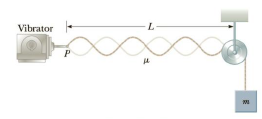Chapter 14, Problem 50P

Chapter
Section
Textbook Problem

In the arrangement shown in Figure P14.50, an object of mass m = 5.0 kg hangs from a cord around a light pulley. The length of the cord between point P and the pulley is L = 2.0 m. (a) When the vibrator is set to a frequency of 150 Hz, a standing wave with six loops is formed. What must be the linear mass density of the cord? (b) How many loops (if any) will result if m is changed to 45 kg? (c) How many loops (if any) will result if m is changed to 10 kg?Figure P14.50

(a)

To determine
The linear mass density of the cord for the six loops standing waves.

Explanation

Given Info: From the given figure six loops are formed using that wavelength of the standing waves can be calculated, length of the cord is 2.0m and frequency of the vibrator is 150Hz .

Formula to calculate the wavelength of standing wave formed with six loops is,

6(λ2)=L

• λ is the wavelength of standing wave,
• L is the length of the cord,

Rewrite the relation in terms of λ .

λ=2L6=L3

Substitute 2.0m for L to find λ .

λ=2.0m3=0.67m

The speed of the waves in the string is,

v=λf

• v is the speed of the waves,
• f is the frequency set by the vibrator,

Substitute 0.67m for λ and 150Hz for f.

v=(0.67m)(150Hz)=100.5m/s

Thus, the speed of the waves in the string is 100.5 m/s.

Conclusion:

Formula to calculate the tension in the string is,

F=mg

• F is the tension force,
• m is the mass of the object,
• g is the acceleration due to gravity,

Substitute 5

(b)

To determine
The number of loops formed when the mass has changed to 45 kg.

(c)

To determine
The number of loops formed when the mass has changed to 10 kg.

Still sussing out bartleby?

Check out a sample textbook solution.

See a sample solution

The Solution to Your Study Problems

Bartleby provides explanations to thousands of textbook problems written by our experts, many with advanced degrees!

Get Started

Find more solutions based on key concepts